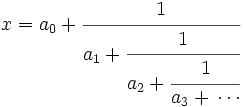## Monday, October 03, 2005

### Continued Fractions

The proof for Fermat's Last Theorem n = 5 depends on Continued Fractions. A Continued Fraction is an expression of the following form:where a0, a1, etc. are all integers. To simplify this characterization, the Continued Fraction is represented using the following notation:
[ a0, a1, a2, ... ]

To understand the power of continued fractions, let's look at how they can be used to represent the square root of 2. Being an irrational number, the digits that make up the real number do not repeat (if they repeated, then it would not be an irrational number, see here). The digits we get are: 1.4142135...

Using continued fractions, we can represent this same value as [1,2,2,2...] where 2 repeats for ever (see below).

Continued Fractions as an idea have their origin withEuclid's Algorithm for finding the greatest common denominator of two integers. Popular study of Continued Fractions began with the work of John Wallis who was the first to use the term "continued fractions."

Later, Leonhard Euler established the foundations of continued fractions. He demonstrated, for example, that every rational number can be expressed as a terminating, simple continued fraction, established a formula for the constant e in continued fraction form, and used this to prove that e is irrational (more on this in a future blog)

Other important work was done by Joseph Louis Lagrange. He used continued fractions to show the value of irrational roots and proved that the real root of a quadratic irrational is a periodic continued fraction (more on this result in a future blog).

The story of continued fractions goes greatly beyond this short outline. For those interested in understanding the larger story, please check out the references at the bottom of this blog.

First, it should be clear that all real numbers can be represented as continued fractions.

Lemma 1: We can generated a continued fractions of the form [ a0, a1, a2, ... ] for any real number.

(1) Let α be any real number.
(2) Let's define a function floor() such that the floor() of any real number is the highest integer that is lower than the real number. For example floor(π) = 3.
(3) Now, here are the rules for constructing the continued fraction:
(i) Let α0 = α
(ii)Let an = floorn)
(iii) Let αn+1 = 1/(αn - an)
(4) For any integer, the continued fraction is [a0] since floor(any integer) = the integer.
(5) If it is not an integer, then we use (iii) to get α1 and use (ii) to determine a1.
(6) In this way, we can generate a continued fraction for any real value.

QED

As an example, let's use the algorithm above to generate the continued fraction for 2:

Corrolary: √2 = [ 1,2,2,2...]

(1) a0 = floor(√2) = 1.

(2) α1 = 1/(2 - 1) =2 + 1 [By multiplying both sides by 2 + 1 ]

(3) a1 = floor(√2 + 1) = floor(√2) + 1 = 2

(4) α2 = 1/(√2 + 1 - 2) = 1/(√2 - 1) = √2 + 1.

(5) This means that we are at the same value as step (2) which means that the value 2 goes on ad infinitum.

QED

References

#### 1 comment:

Unknown said...

This might already be in a later post on this blog, but it seems worth noting that the continued fraction for the Golden Mean is [1,1....]